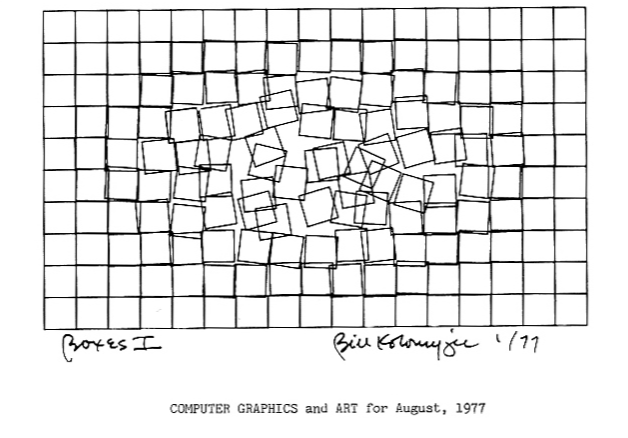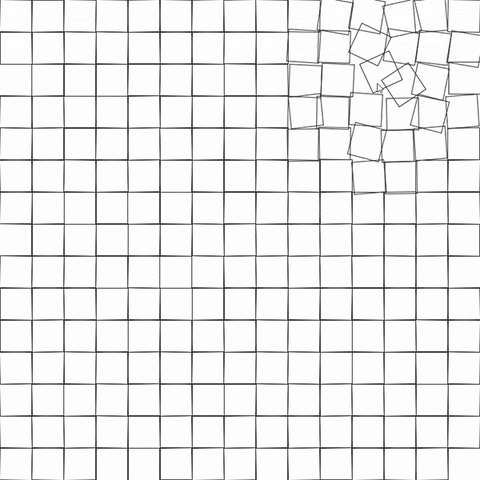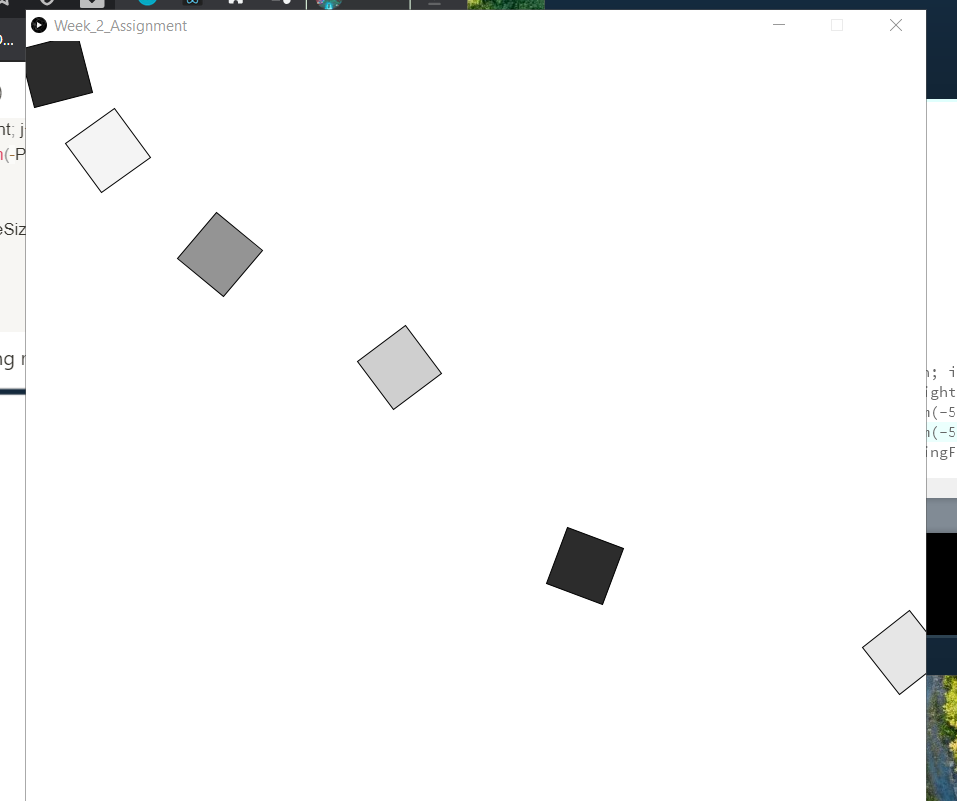# [Week 2] Recreating computer art

The assignment for week 2 involves using `for()` and/or `while()` loops to create a work of art. I looked through the sources of computer arts and they are very fascinating. I particularly like this following art and decided to try and recreate it with Processing.My workflow for this piece is as following:

1. Create the basic construction of grid-like squares (which makes good use of loops)
2. Add interaction by randomizing the movement of the squares according to mouse movement
3. Extra: experiment with colors

My final product is the following and the code can be found at the end of this post:Basic construction

The grid-like foundation of the art is pretty straightforward and problem-free. I use two nested `for()` loops to draw the rectangles.

Interactive movement

To create the randomized movements of the squares, I make extensive use of the `random()` function.

Randomizing the shifting of the squares (moving slightly in the horizontal and vertical direction) is also straightforward, but I ran into an issue when trying to slightly rotate the squares. The `rotate()` function is usually used in tandem with `translate()` because the shape will be rotated relative to the origin. The problem is that `translate()` only gets reset after each loop of `draw()`, so when I want to rotate all of the squares within a single `draw()` loop, the positions of the squares are mixed up because they get translated further and further from the initial origin.I found that a good solution to this issue is to use the pair of functions `pushMatrix()` and `popMatrix()`. Using these two functions to enclose `translate()` and `rotate()` ensures that the coordinate system is restored to its original state before the next square is drawn, making sure all the squares are correctly positioned and rotated.

I also want to have some user interaction with the art so that as the user moves the mouse around the canvas, the closest squares to the mouse will have greatest movements. For this, I use the `dist()` function to calculate how far a square is from the current mouse position and give each square a `distFactor` based on the calculated distance. This `distFactor` will then be used to calculate `rotatingFactor` and `movingFactor`s to decide the movements of the squares. With this, the piece of art is finished.

Extra: Coloring

I add an extra function to the code so that when the user clicks on the canvas, coloring mode will be on and instead of black border with no fill, the squares will have no border and random colors. The coloring mode part has nothing to do with recreating the original art; I just want to experiment around with `random()` and colors.

Code

```int numOfSquares = 15;
int squareSize;
float movingRange;
boolean colorOn = false;

void setup() {
frameRate(10);

size(900, 900);
squareSize = width/numOfSquares;
movingRange = squareSize*.1;
rectMode(CENTER);
}

void draw() {
background(255);

for (int i=squareSize/2; i<width; i+=squareSize) {
for (int j=squareSize/2; j<height; j+=squareSize) {
float distance = dist(i, j, mouseX, mouseY);
float distFactor = 1/distance * 100;
distFactor *= .01;
}
float rotatingFactor = random(-PI/10, PI/10) * distFactor;
float movingFactorX = random(-movingRange, movingRange) * distFactor;
float movingFactorY = random(-movingRange, movingRange) * distFactor;

if (colorOn) {
noStroke();
color squareColor = color(random(0, 255), random(0, 255), random(0, 255));
float alpha = map(distance, 0, width, 0, 255/2);
fill(squareColor, alpha + random(-10, 10));
}
else {
noFill();
stroke(0);
}
pushMatrix();
translate(i+movingFactorX, j+movingFactorY);
rotate(rotatingFactor);
rect(0, 0, squareSize, squareSize);
popMatrix();
}
}
}

void mouseClicked() {
colorOn = !colorOn;
}```